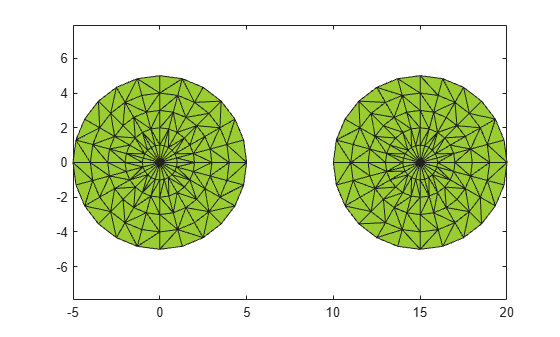inShape

Determine if point is inside alpha shape

Description

example

tf = inShape(shp,qx,qy) returns logical 1 (true) values for the 2-D query points (qx,qy) that are within 2-D alpha shape shp. Otherwise, inShape returns values of logical 0 (false). The qx and qy arguments are numeric arrays whose corresponding elements specify the (x,y) query point coordinates.

tf = inShape(shp,qx,qy,qz) tests whether the 3-D query points (qx,qy,qz) are within 3-D alpha shape shp.

tf = inShape(shp,QP) specifies the 2-D or 3-D query point coordinates in a matrix with 2 or 3 columns.

tf = inShape(___,RegionID) tests whether the query points are within a specific region of the alpha shape, using any of the previous syntaxes. RegionID is the ID for the region and 1RegionIDnumRegions(shp).

[tf,ID] = inShape(___) also returns the IDs for the regions in the alpha shape that contain the query points. ID is NaN for query points that are not in the alpha shape.

Examples

collapse all

Create a set of 2-D points.

th = (pi/12:pi/12:2*pi)';
x1 = [reshape(cos(th)*(1:5), numel(cos(th)*(1:5)),1); 0];
y1 = [reshape(sin(th)*(1:5), numel(sin(th)*(1:5)),1); 0];
x = [x1; x1+15;];
y = [y1; y1];

Create and plot an alpha shape using an alpha radius of 2.5.

shp = alphaShape(x,y,2.5);
plot(shp)Create a Cartesian grid of query points near the alpha shape.

[qx, qy] = meshgrid(-10:2:25, -10:2:10);

Check if the query points are inside of the alpha shape, and if so, plot them red. Plot the query points that lie outside of the alpha shape in blue.

in = inShape(shp,qx,qy);
plot(shp)
hold on
plot(qx(in),qy(in),'r.')
plot(qx(~in),qy(~in),'b.')Input Arguments

collapse all

Alpha shape, specified as an alphaShape object. For more information, see alphaShape.

Example: shp = alphaShape(x,y) creates a 2-D alphaShape object from the (x,y) point coordinates.

Query point x-coordinates, specified as a numeric array.

Data Types: double

Query point y-coordinates, specified as a numeric array.

Data Types: double

Query point z-coordinates, specified as a numeric array.

Data Types: double

Query point coordinates, specified as a matrix with two columns (2-D) or a matrix with three columns (3-D).

• For 2-D, the columns of QP represent x and y coordinates, respectively.

• For 3-D, the columns of QP represent x, y, and z coordinates, respectively.

Data Types: double

ID number for region in alpha shape, specified as a positive integer scalar between 1 and numRegions(shp).

An alpha shape can contain several smaller regions, depending on the point set and parameters. Each of these smaller regions is assigned a unique RegionID, which numbers the regions from the largest area or volume to the smallest. For example, consider a 3-D alpha shape with two regions. The region with the largest volume has a RegionID of 1, and the smaller region has a RegionID of 2.

Example: shp.RegionThreshold = area(shp,numRegions(shp)-2); suppresses the two smallest regions in 2-D alpha shape shp.

Data Types: double

Output Arguments

collapse all

Status of the query points, returned as a logical array. The size of tf is equal to the size of the inputs that specify the query points (qx, qy, qz, or QP).

inShape returns logical 1 (true) values for points that are within the alpha shape or exactly on the boundary.

IDs of regions containing query points, returned as a numeric array. ID is the same size as tf.